# Mathematics facts for kids

Kids Encyclopedia Facts
(Redirected from Maths)

Mathematics is the study of numbers, shapes and patterns. The word comes from the Greek word "μάθημα" (máthema), meaning "science, knowledge, or learning", and is sometimes shortened to maths (in England, Australia, Ireland, and New Zealand) or math (in the United States and Canada). The short words are often used for arithmetic, geometry or simple algebra by students and their schools.

Mathematics includes the study of:

• Numbers: how things can be counted.
• Structure: how things are organized. This subfield is usually called algebra.
• Place: where things are and their arrangement. This subfield is usually called geometry.
• Change: how things become different. This subfield is usually called analysis.

Mathematics is useful for solving problems that occur in the real world, so many people besides mathematicians study and use mathematics. Today, some mathematics is needed in many jobs. People working in business, science, engineering, and construction need some knowledge of mathematics.

## Problem-solving in mathematics

Mathematics solves problems by using logic. One of the main tools of logic used by mathematicians is deduction. Deduction is a special way of thinking to discover and prove new truths using old truths. To a mathematician, the reason something is true (called a proof) is just as important as the fact that it is true, and this reason is often found using deduction. Using deduction is what makes mathematics thinking different from other kinds of scientific thinking, which might rely on experiments or on interviews.

Logic and reasoning are used by mathematicians to create general rules, which are an important part of mathematics. These rules leave out information that is not important so that a single rule can cover many situations. By finding general rules, mathematics solves many problems at the same time as these rules can be used on other problems. These rules can be called theorems (if they have been proved) or conjectures (if it is not known if they are true yet). Most mathematicians use non-logical and creative reasoning in order to find a logical proof.

Sometimes, mathematics finds and studies rules or ideas that we don't understand yet. Often in mathematics, ideas and rules are chosen because they are considered simple or neat. On the other hand, sometimes these ideas and rules are found in the real world after they are studied in mathematics; this has happened many times in the past. In general, studying the rules and ideas of mathematics can help us understand the world better. Some examples of math problems are addition, subtraction, multiplication, division, calculus, fractions and decimals. Algebra problems are solved by evaluating certain variables. A calculator answers every math problem in the four basic arithmetic operations.

## Areas of study in mathematics

### Number

Mathematics includes the study of numbers and quantities.It is a branch of science the deals with logic of shape,quantity and arrangement. Most of the areas listed below are studied in many different fields of mathematics, including set theory and mathematical logic. The study of number theory usually focuses more on the structure and behavior of the integers rather than on the actual foundations of numbers themselves, and so is not listed in this given subsection.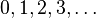$0, 1, 2, 3, \ldots$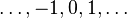$\ldots, -1, 0, 1, \ldots$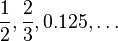$\frac{1}{2}, \frac{2}{3}, 0.125,\ldots$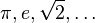$\pi, e, \sqrt{2},\ldots$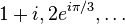$1+i, 2e^{i\pi/3},\ldots$ Natural numbers Integers Rational numbers Real numbers Complex numbers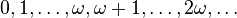$0, 1, \ldots, \omega, \omega + 1, \ldots, 2\omega, \ldots$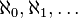$\aleph_0, \aleph_1, \ldots$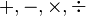$+,-,\times,\div$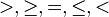$>,\ge, =, \le, <$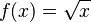$f(x) = \sqrt x$ Ordinal numbers Cardinal numbers Arithmetic operations Arithmetic relations Functions, see also special functions

### Structure

Many areas of mathematics study the structure that an object has. Most of these areas are part of the study of algebra.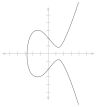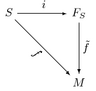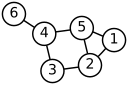Number theory Abstract algebra Linear algebra Order theory Graph theory

### Shape

Some areas of mathematics study the shapes of things. Most of these areas are part of the study of geometry.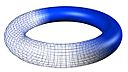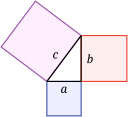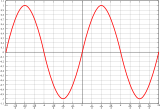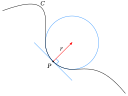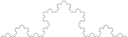Topology Geometry Trigonometry Differential geometry Fractal geometry

### Change

Some areas of mathematics study the way things change. Most of these areas are part of the study of analysis.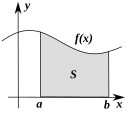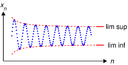Calculus Vector calculus Analysis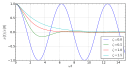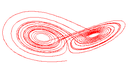Differential equations Dynamical systems Chaos theory

### Applied mathematics

Applied mathematics uses mathematics to solve problems of other areas such as engineering, physics, and computing.
Numerical analysisOptimizationProbability theoryStatistics – Mathematical finance – Game theoryMathematical physicsFluid dynamics - computational algorithms

## Famous theorems

These theorems have interested mathematicians and people who are not mathematicians.

Pythagorean theoremFermat's last theoremGoldbach's conjectureTwin Prime ConjectureGödel's incompleteness theoremsPoincaré conjectureCantor's diagonal argumentFour color theorem – Zorn's lemma – Euler's IdentityChurch-Turing thesis

These are theorems and conjectures that have greatly changed mathematics.

Riemann hypothesisContinuum hypothesisP Versus NPPythagorean theoremCentral limit theoremFundamental theorem of calculusFundamental theorem of algebraFundamental theorem of arithmetic – Fundamental theorem of projective geometry – classification theorems of surfaces – Gauss-Bonnet theoremFermat's last theorem - Kantorovich theorem

## Foundations and methods

Progress in understanding the nature of mathematics also influences the way mathematicians study their subject.

Philosophy of Mathematics – Mathematical intuitionism – Mathematical constructivism – Foundations of mathematics – Set theory – Symbolic logic – Model theory – Category theoryLogic – Reverse Mathematics – Table of mathematical symbols

### History and the world of mathematicians

Mathematics in history, and the history of mathematics.

History of mathematics – Timeline of mathematics – MathematiciansFields medalAbel PrizeMillennium Prize Problems (Clay Math Prize) – International Mathematical Union – Mathematics competitions – Lateral thinking – Mathematics and gender

## Awards in mathematics

There is no Nobel prize in mathematics. Mathematicians can receive the Abel prize and the Fields Medal for important works.

The Clay Mathematics Institute has said it will give one million dollars to anyone who solves one of the Millennium Prize Problems.

## Mathematical tools

There are many tools that are used to do mathematics or to find answers to mathematics problems.

Older tools